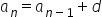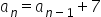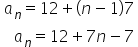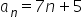Maths-
General
Easy

Question

# Write recursive formula and explicit formula. 12,19,26,33,40,...Hint:

## The correct answer is: a_n=7n+5.

### Explanation: We have given a sequence 12,19,26,33,40,.... We have to find the recursive and explicit formula of the given sequence. Step 1 of 2:We have given a sequence 12,19,26,33,40,....The given sequence is an AP.We know that the recursive formula for any AP is, where d is common difference.Here the common difference is 7So, The recursive formula isStep 2 of 2:The given sequence is an AP.So, The explicit formula will be.#### With Turito Foundation.#### Get an Expert Advice From Turito.